# Arrays, Multiplication and Division

##### Age 5 to 11

Published 2012 Revised 2021

Arrays, multiplication and division

Jennie Pennant, with the help of Jenni Way and Mike Askew, explores how the array can be used as a thinking tool to help children develop an in-depth understanding of multiplication and division.

Using Arrays to Explore Numbers

Arrays are useful models for multiplication which can be used in a variety of ways, ranging from highly structured lessons to games and open investigations.

An array is formed by arranging a set of objects into rows and columns. Each column must contain the same number of objects as the other columns, and each row must have the same number as the other rows.

The following array, consisting of four columns and three rows, could be used to represent the number sentence 3 x 4 = 12, 4 x 3 =12, 3 + 3 + 3 + 3 = 12 and 4 + 4 + 4 =12.Building Multiplication Facts and Tables

Arrays can be used for building multiplication facts in a meaningful way. Before drilling and memorising tables, children must understand how these facts are derived. For example, by progressively adding another column of three objects, children can build the three-times tables for themselves. This representation not only assists in understanding the process, but provides a visual image for children to draw upon as they begin to use and memorise the basic number facts.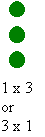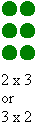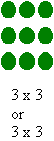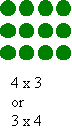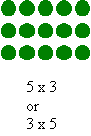Using arrays to explore larger numbers

Arrays can be helpfully used to explore calculations such as 13 x 5 where the array can be split into useful chunks such as 10 and 3. This means that children can use their known number facts to work out calculations.

Here 13 x 5 = (10 x 5) + (3 x 5).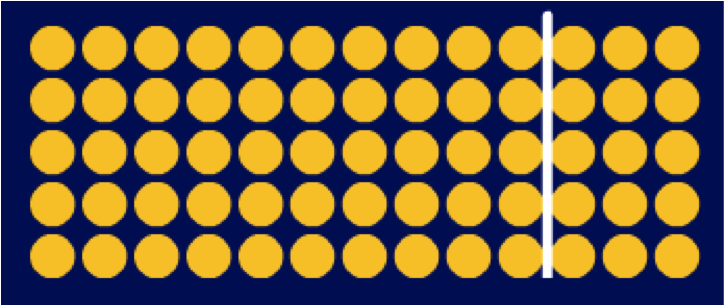After a while drawing all the dots can get very tedious! The blank array then becomes a very useful tool for helping children model their thinking and work out how to do more complex multiplications in an informal way.

Here's a child using the blank array, as a thinking tool, to help them work out 15 x 14.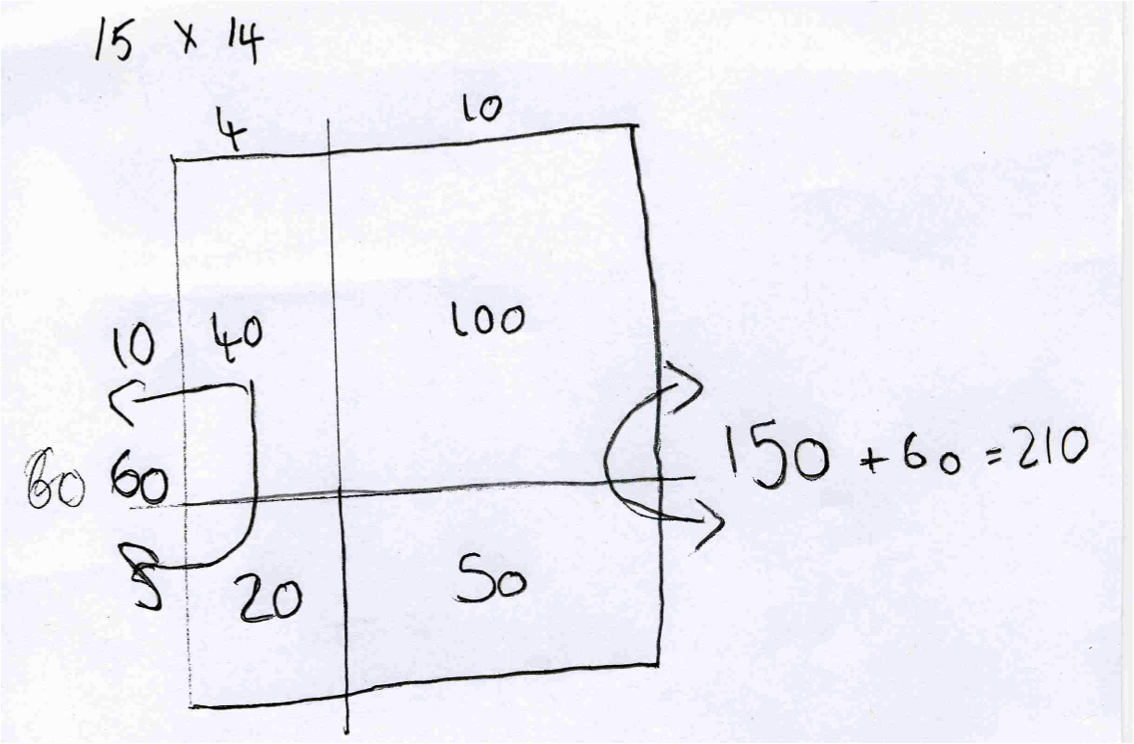The blank array helps children to use other strategies, such as compensating, when carrying out multiplication. Here, to work out 34 x 9, the child has decided to do 34 x 10 and then take off the 34 x 1.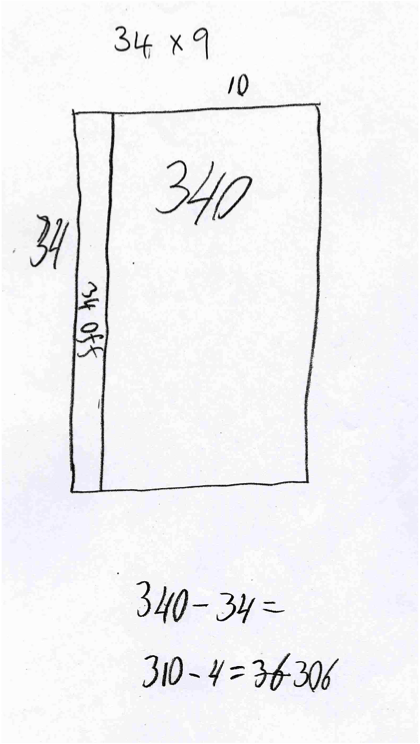Beyond the blank array this 'dividing the multiplication into easy parts' strategy can be formalised into the grid method. The children can see how the 'abstract' grid method overlays the array and formalises the blank array into a standard form.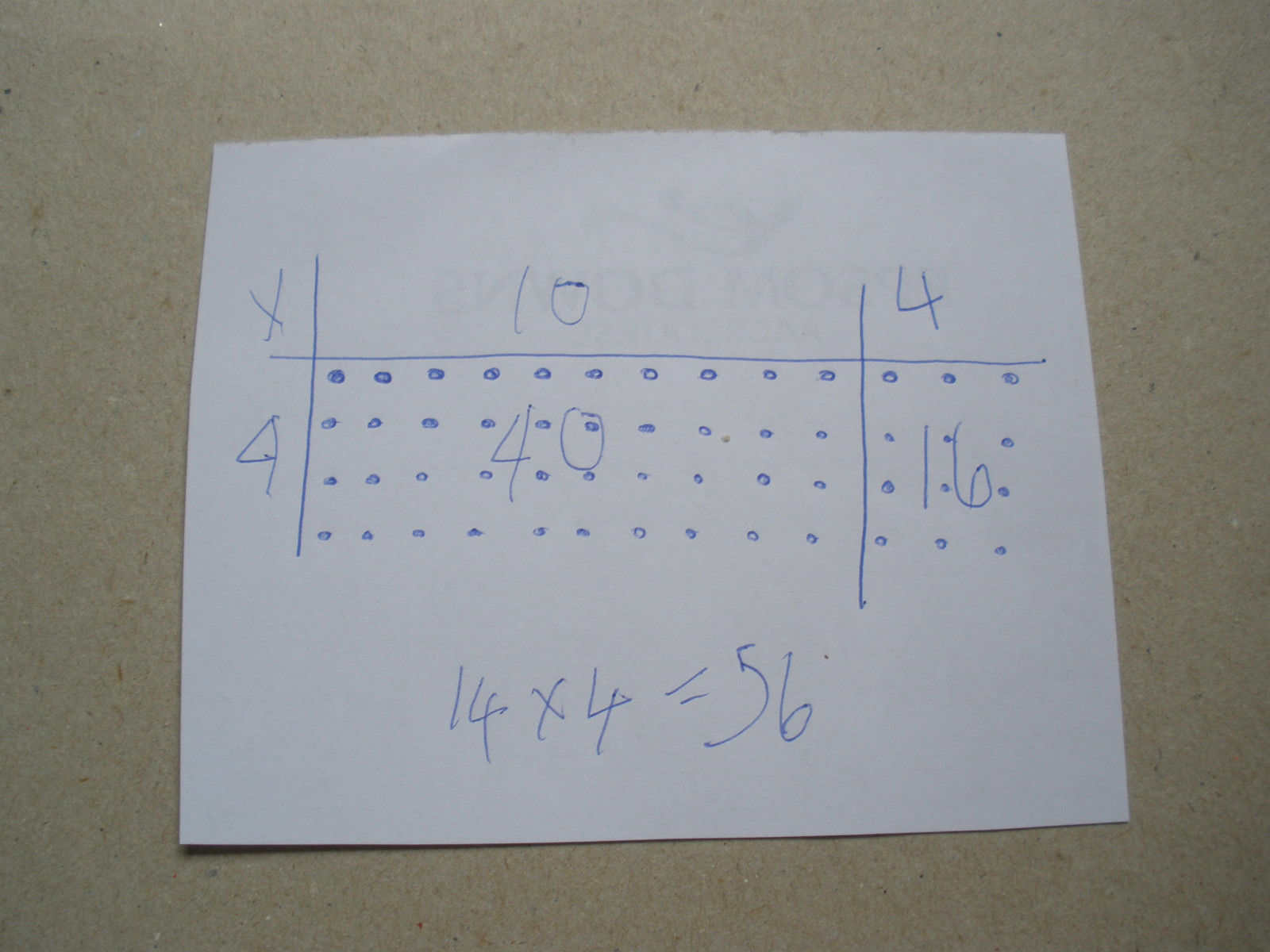Division as the Inverse Operation of Multiplication

Of the four operations, division is the most troublesome for young students. Full understanding of division tends to lag well behind the other operations. For many children opportunities to explore the concept with concrete materials are curtailed well before they perceive the relationships between division and the other three operations. One such relationship, the inverse relationship between division and multiplication, can be effectively illustrated using arrays.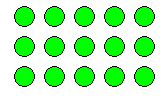For example; 3×5=15  or 3 rows of 5 make 15, can be represented by the following array.

Looking at the array differently reveals the inverse, that is
15÷3=5  or 15 put into 3 rows makes 5 columns - or 5 in each row.

Language clearly plays an important role in being able to express the mathematical relationships and the physical array supports this aspect of understanding by giving the children a concrete image to talk about.

Placing the mathematics into a real-life context through word problems can facilitate both understanding of the relationship and its expression through words.

For example, "The gardener planted 3 rows of 5 seeds. How many seeds did she plant?" poses quite a different problem to "The gardener planted 15 seeds in 3 equal rows. How many seeds in each row?" yet both these word problems can be modelled using the same array.

Further exploration of the array reveals two more ways of expressing inverse relationships: 5×3=15 and 15÷3=5 .

The word problems can be adapted to describe these operations and highlight the similarities and differences between the four expressions modelled by the one array.

Using the blank array
Suppose you want to figure out 176 ÷ 8. We can set this up as an array with the value of one side missing.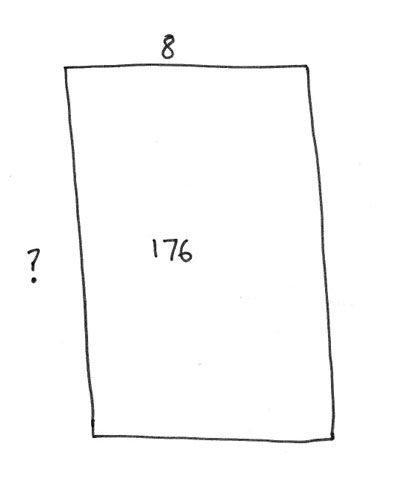Using known multiplication facts the value of the missing side can be built up.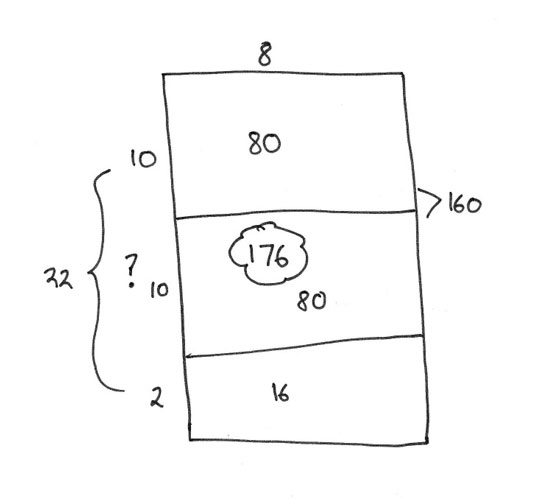So the child can see that 22 lots of 8 is the same as 176.

The array is a very powerful tool for supporting the development of children's thinking around both multiplication and division.